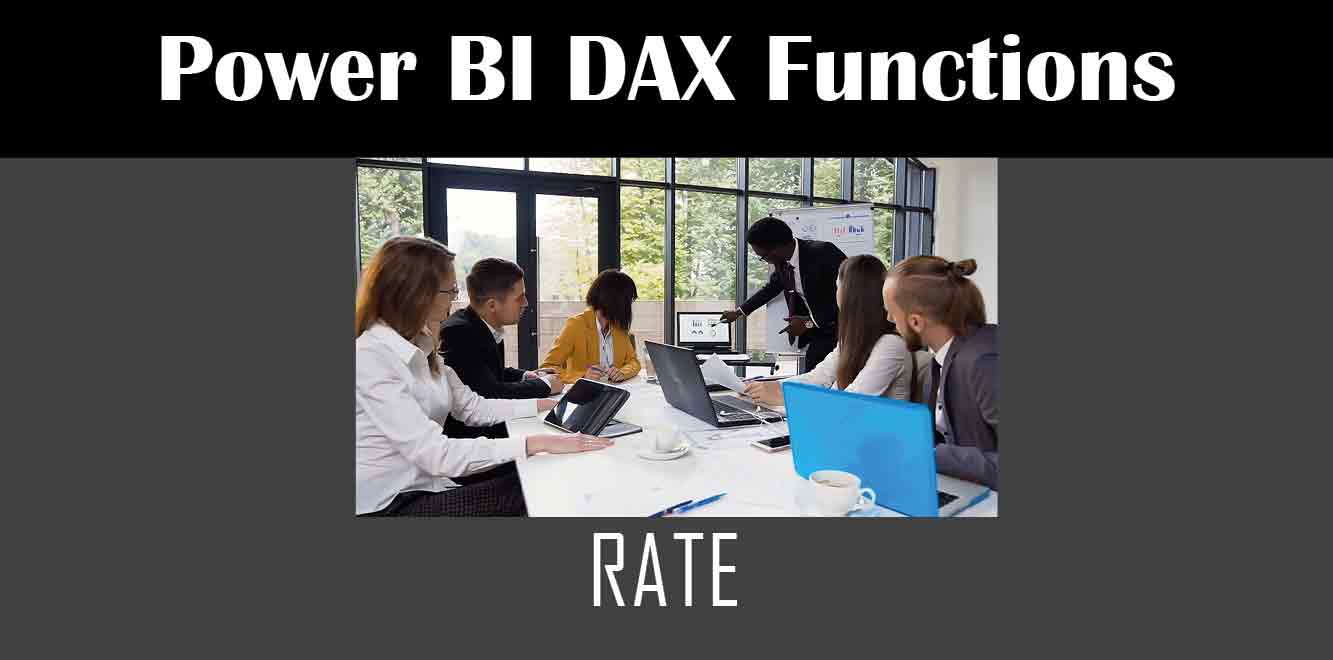# RATE

## Y## What is the RATE Function?

The RATE function is a financial function in Power BI that helps you calculate the interest rate of a loan or an investment. You can use this function to find out the interest rate that a borrower needs to pay on a loan or the return that an investor can expect on an investment.

## Syntax of the RATE Function

The syntax of the RATE function in Power BI is as follows:

`RATE(nper, pmt, pv, [fv], [type], [guess])`

Here is what each parameter means:

– nper: The total number of payment periods in an investment or a loan.

– pmt: The payment made each period in an investment or a loan.

– pv: The present value of an investment or a loan.

– fv: The future value of an investment or a loan. This parameter is optional.

– type: The timing of the payment. This parameter is optional.

– guess: An estimate for the interest rate. This parameter is optional.

## How to Use the RATE Function in Power BI

To use the RATE function in Power BI, you need to follow these steps:

1. Open Power BI and create a new report.

2. Click on the “New Measure” button in the “Modeling” tab.

3. Enter a name for your measure and type the following formula:

Rate = RATE(nper, pmt, pv, [fv], [type], [guess])

``` 4. Replace the values of the parameters with the values of your investment or loan. 5. Press Enter to save the formula. Once you have created the measure, you can use it in your report to calculate the interest rate of your investment or loan. Examples of Using the RATE Function Here are some examples of using the RATE function in Power BI: Example 1: Calculating the Interest Rate of a Loan Suppose you borrowed \$10,000 from a bank and you need to pay it back in five years with an annual interest rate of 5%. You can use the RATE function to calculate the monthly interest rate that you need to pay. Here is the formula: ```

Rate = RATE(5*12, -10000/60, 10000, , 0, 0.05/12)

``` In this formula, we have used the following values: - nper: 5*12 (since there are five years with 12 payments each year). - pmt: -10000/60 (since you need to pay back \$10,000 in 60 months). - pv: 10000 (since you borrowed \$10,000). - fv: The default value of 0 (since there is no future value). - type: 0 (since the payments are made at the end of each period). - guess: 0.05/12 (since the annual interest rate is 5% and we need to calculate the monthly rate). The result of this formula is 0.001983, which is the monthly interest rate that you need to pay. Example 2: Calculating the Return of an Investment Suppose you invested \$10,000 in a stock and you expect to receive \$12,000 after three years. You can use the RATE function to calculate the annual return that you can expect from this investment. Here is the formula: ```

Rate = RATE(3, , -10000, 12000, 0, 0.1)

``` In this formula, we have used the following values: - nper: 3 (since the investment lasts three years). - pmt: The default value of 0 (since there are no payments made). - pv: -10000 (since you invested \$10,000). - fv: 12000 (since you expect to receive \$12,000 after three years). - type: 0 (since there are no payments made). - guess: 0.1 (since we estimate the annual return to be 10%). The result of this formula is 0.035744, which is the annual return that you can expect from this investment. In this article, we have discussed how to use the Power BI DAX function RATE to calculate the interest rate of a loan or an investment. We have also provided examples of using this function in different scenarios. By using the RATE function in Power BI, you can make better financial decisions and improve your investment strategy. Power BI DAX Training Courses by G Com Solutions (0800 998 9248)Power BI DAX Intensive Training Course £1,050.00 – £26,550.00 Select optionsContinue Loading DonePower BI DAX Introduction £395.00 – £9,750.00 Select optionsContinue Loading DonePower BI DAX Intermediate £395.00 – £9,750.00 Select optionsContinue Loading DonePower BI DAX Advanced £395.00 – £9,750.00 Select optionsContinue Loading Done Upcoming Courses 24-26 Oct 23 (London or Online) Contact Us Subject Your Name (required) Company/Organisation Email (required) Telephone Training Course(s) Power BI Intensive TrainingPower BI introduction Power BI IntermediatePower BI AdvancedDAXPower Query MPower BI CertificationPower BI AdministrationPower PlatformPower AutomatePower AppsOTHER Your Message Upload Example Document(s) (Zip multiple files) ```
``` ```
``` ```
``` ```
``` ```
``` ```